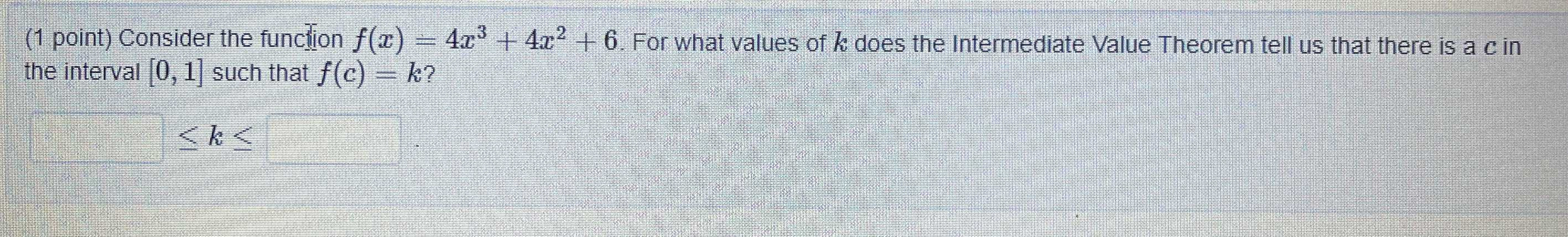### ¿Todavía tienes preguntas de matemáticas?

Pregunte a nuestros tutores expertos
Algebra
Pregunta(1 point) Consider the function $$f ( x ) = 4 x ^ { 3 } + 4 x ^ { 2 } + 6$$ . For what values of $$k$$ does the Intermediate Value Theorem tell us that there is a $$c$$ in the interval $$[ 0,1 ]$$ such that $$f ( c ) = k ?$$

$$\begin{pmatrix} \end{pmatrix}$$$$\leq k \leq$$ $$\begin{pmatrix} \end{pmatrix}$$

6$$\le$$k$$\le$$14